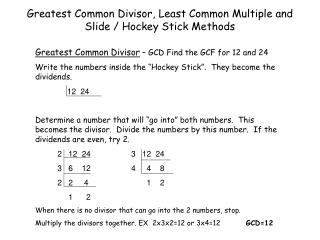DownloadDownload PresentationGreatest Common Divisor, Least Common Multiple and Slide / Hockey Stick Methods

# Greatest Common Divisor, Least Common Multiple and Slide / Hockey Stick Methods

Download Presentation## Greatest Common Divisor, Least Common Multiple and Slide / Hockey Stick Methods

- - - - - - - - - - - - - - - - - - - - - - - - - - - E N D - - - - - - - - - - - - - - - - - - - - - - - - - - -
##### Presentation Transcript

1. Greatest Common Divisor, Least Common Multiple and Slide / Hockey Stick Methods Greatest Common Divisor – GCD Find the GCF for 12 and 24 Write the numbers inside the “Hockey Stick”. They become the dividends. 12 24 Determine a number that will “go into” both numbers. This becomes the divisor. Divide the numbers by this number. If the dividends are even, try 2. 2 12 24 3 12 24 3 6 12 4 4 8 2 2 4 1 2 1 2 When there is no divisor that can go into the 2 numbers, stop. Multiply the divisors together. EX 2x3x2=12 or 3x4=12 GCD=12

2. Least Common Multiple – LCM Find the LCM for 12 and 24 • The least common multiple is found by following the steps above. Once you have finished the “Hockey Stick”, multiply all of the outside numbers together (horizontally and vertically). The result is the LCM for the beginning 2 numbers. In the above example, multiply • 2x3x2x2=24 or 3x4x2=24 • Simplifying Fractions – ex 24/96 • Write the numerator and the denominator in the “Hockey Stick”. • Proceed with the slide method until no number can go into the 2 numbers. • The resulting two numbers are the new numerator and denominator. • 24 96 5 15 75 • 8 32 3 3 15 • 2 8 1 5 • 1 4 • 24/96 =1/4 15/75 =1/5